# A Study of the Thermo-Mechanical Behavior of a Gas Turbine Blade in Composite Materials Reinforced with Mast

A Study of the Thermo-Mechanical Behavior of a Gas Turbine Blade in Composite Materials Reinforced with Mast
Laboratoire de Recherche en Productique (LRP), Department of Mechanics, University Batna 2 (Mostefa Ben Boulaïd), Batna 05000, Algeria

Laboratoire Systems Electro-mécanique (LSELM), Department of Electro-mechanics, University Badji-Mokhtar Annaba, Annaba 02300, Algeria

Laboratory of Innovation in Construction, Eco-design, and Seismic Eng. (LICEGS) University of Batna 2 (Mostefa Ben Boulaïd), Batna 05000, Algeria

Corresponding Author Email:
f.khalid@univ-batna2.dz
Page:
101-108
|
DOI:
https://doi.org/10.18280/rcma.310205
10 December 2019
|
Accepted:
21 October 2020
|
Published:
30 April 2021
| Citation

OPEN ACCESS

Abstract:

The turbine blades are subjected to high operating temperatures and high centrifugal tensile stress due to rotational speeds. The maximum temperature at the inlet of the turbine is currently limited by the resistance of the materials used for the blades. The present paper is focused on the thermo-mechanical behavior of the blade in composite materials with reinforced mast under two different types of loading. The material studied in this work is a composite material, the selected matrix is a technical ceramic which is alumina (aluminum oxide Al2O3) and the reinforcement is carried out by short fibers of high modulus carbon to optimize a percentage of 40% carbon and 60% of ceramics. The simulation was performed numerically by Ansys (Workbench 16.0) software. The comparative analysis was conducted to determine displacements, strains and Von Mises stress of composite material and then compared to other materials such as Titanium Alloy, Stainless Steel Alloy, and Aluminum 2024 Alloy. The results were compared in order to select the material with the best performance in terms of rigidity under thermo-mechanical stresses. While comparing these materials, it is found that composite material is better suited for high temperature applications. On evaluating the graphs drawn for, strains and displacements, the blade in composite materials reinforced with mast is considered as optimum.

Keywords:

alumina, blade, thermo-mechanical behavior, Ansys, short fibers, high modulus carbon, speeds

1. Introduction

2. Materials and Methods

To observe the thermo-mechanical behavior of blade in composite material, in this study, we will calculate the displacements and the deformations of the blade in composite material with random orientation and super-alloy. The characteristics of the gas turbine blade manufacturing materials are listed in Table 1 and 2.

Table 1. Mechanical and thermal characteristics of super alloys 

 Materials Titanium Alloy Stainless Steel Alloy Aluminum 2024 Alloy Density [Kg/m3] 4700 8025 2750 Young’s modulus [GPa] 205 200 73 Poisson’s ratio 0.33 0.30 0.33 Yield strength [MPa] 1000 1050 470 Coefficient of thermal expansion 10-6℃ 8.8 14.5 23.3

Table 2. Mechanical and thermal characteristics of high modulus carbon (HM) and alumina 

 Materials Carbon (HM) Alumina (Al2O3) Density [Kg/m3] 1800 3700 Young’s modulus [GPa] 390 380 Poisson’s ratio 0.35 0.25 Yield strength [MPa] 2500 1400 Coefficient of thermal expansion $\left[10^{-6}\left({ }^{\circ} \mathrm{C}^{-1}\right)\right]$ 0.8 8
3. Determination of Composite Mechanical and Thermal Characteristics

3.1 Mechanical characteristics

All equations of mechanical characteristics are given by Gay  and are recalled here for more clearness.

Longitudinal modulus of elasticity EL is given by the following equation:

$\mathrm{E}_{\mathrm{L}}=\mathrm{E}_{\mathrm{f}} V_{\mathrm{f}}+\mathrm{E}_{\mathrm{m}}\left(1-\mathrm{V}_{\mathrm{f}}\right)$     (1)

Transverse modulus of elasticity ET is expressed as follows:

$\mathrm{E}_{\mathrm{T}}=\frac{\mathrm{E}_{\mathrm{m}} \mathrm{E}_{\mathrm{f}}}{\mathrm{E}_{\mathrm{f}} \mathrm{V}_{\mathrm{m}}+\mathrm{E}_{\mathrm{m}} \mathrm{V}_{\mathrm{f}}}$     (2)

Then Poisson’s ratio νL and $v_{\mathrm{T}}$ is written as:

$\mu_{\mathrm{L}}=v_{\mathrm{f}} V_{\mathrm{m}}+v_{\mathrm{m}}\left(1-V_{\mathrm{f}}\right)$     (3)

$\mu_{\mathrm{T}}=\mu_{\mathrm{L}} \frac{\mathrm{E}_{\mathrm{T}}}{\mathrm{E}_{\mathrm{L}}}$     (4)

Finally the Longitudinal shear modulus GL and Transverse shear modulus GT expressed as follows:

$\mathrm{G}_{\mathrm{L}}=\frac{\mathrm{G}_{\mathrm{m}} \mathrm{G}_{\mathrm{f}}}{\mathrm{G}_{\mathrm{f}} \mathrm{V}_{\mathrm{m}}+\mathrm{G}_{\mathrm{m}} \mathrm{V}_{\mathrm{f}}}$   (5)

$G_{T}=\frac{E_{T}}{2\left(1+v_{T}\right)}$     (6)

3.2 Thermal characteristics

Thermal characteristics are explained and resumed by Mallick .

The longitudinal thermal expansion $\alpha_{\mathrm{L}} \text { and }$ Transverse thermal expansion are written as:

$\alpha_{\mathrm{L}}=\frac{\left(1-\mathrm{V}_{\mathrm{f}}\right) \alpha_{\mathrm{m}} \mathrm{E}_{\mathrm{m}}+\mathrm{V}_{\mathrm{f}} \alpha_{\mathrm{f}} \mathrm{E}_{\mathrm{f}}}{\left(1-\mathrm{V}_{\mathrm{f}}\right) \mathrm{E}_{\mathrm{m}}+\mathrm{V}_{\mathrm{f}} \mathrm{E}_{\mathrm{f}}}$     (7)

$\alpha_{\mathrm{T}}=\left(1-\mathrm{V}_{\mathrm{f}}\right) \alpha_{\mathrm{m}}+\mathrm{V}_{\mathrm{f}} \alpha_{\mathrm{f}}+\left(\alpha_{\mathrm{f}}-\alpha_{\mathrm{m}}\right) \frac{\mathrm{v}_{\mathrm{f}} \mathrm{E}_{\mathrm{m}}-\mathrm{v}_{\mathrm{m}} \mathrm{E}_{\mathrm{f}}}{\frac{\mathrm{E}_{\mathrm{m}}}{\mathrm{V}_{\mathrm{f}}}+\frac{\mathrm{E}_{\mathrm{f}}}{1-\mathrm{V}_{\mathrm{f}}}}$    (8)

3.3 Mechanical properties of the composite with mast reinforcement 

In this case the corresponding transformed reduced rigidity will be examined by:

$\widetilde{Q}_{i j}=\frac{\int_{0}^{2 \pi}\left(\bar{Q}_{i j}\right) \partial \theta}{\int_{0}^{2 \pi} \partial \theta}$    (9)

And the elastic modules of the mast layer are:

$\mathrm{E}_{\mathrm{mât}}=\frac{\left(\mathrm{V}_{1}-\mathrm{V}_{4}\right)\left(\mathrm{V}_{1}+\mathrm{V}_{4}\right)}{\mathrm{V}_{1}}$

$v_{\text {mât }}=\frac{V_{4}}{V_{1}}$     (10)

$G_{\text {mât }}=\frac{V_{1}-V_{4}}{2}$

With the expressions of $\mathrm{V}_{\mathrm{i}}$ are:

$V_{1}=\frac{1}{8}\left(3 Q_{11}+3 Q_{22}+3 Q_{12}+4 Q_{66}\right)$;

$V_{2}=\frac{1}{2}\left(Q_{11}-Q_{22}\right)$;     (11)

$V_{3}=\frac{1}{8}\left(Q_{11}+Q_{22}-2 Q_{12}\right)$;

$\mathrm{V}_{4}=\frac{1}{8}\left(\mathrm{Q}_{11}+\mathrm{Q}_{22}+6 \mathrm{Q}_{12}-4 \mathrm{Q}_{66}\right)$

4. Choice of Composite Materials for the Realization of the Blade

In our study we propose to realize a gas turbine blade in composite materials with reinforced mast. The material studied is a composite material, the selected matrix is a technical ceramic which is an alumina (aluminum oxide Al2O3) and the reinforcement is carried out by short fibers of carbon HM to optimize a percentage of 40% carbon and 60% of ceramics.

5. Characteristics of the Composite Material

5.1 By the law of mixtures

The characteristics of composite material studied are presented below in Tables 3 and 4, respectively.

Table 3. Mechanical and thermal characteristics of the material studied

 Young’s Modulus [GPa] EL 384 ET 383.94 Poisson’s ratio $\boldsymbol{v}_{\mathrm{L}}$ 0.29 $\mathbf{v}_{\mathrm{T}}$ 0.29 Shear modulus [GPa] $\mathbf{G}_{\mathrm{L}}$ 148.88 $\mathbf{G}_{\mathrm{T}}$ 148.82 thermal expansion  [ $\left[10^{-6}\left({ }^{\circ} \mathrm{C}^{-1}\right)\right]$ ] $\alpha_{\mathrm{L}}$ 5.075 $\alpha_{\mathrm{T}}$ 4.96

5.2 By the law of the behavior of the composite with reinforcement mast

Table 4. Mechanical properties of the mast reinforced composite

 $\text { Young's Modulus }$ $\mathbf{E}_{\mathbf{m â t}}[\mathrm{GPa}]$ $\text { Poisson's ratio }$ $\mathbf{v}_{\mathbf{m â t}}$ $\text { Shear modulus }$ $\mathbf{G}_{\mathbf{m â t}}[\mathrm{GPa}]$ 400.44 0.2797 156.45
6. Numerical Analysis

Three-dimensional model of the gas turbine blade is first modeled using the solid works software as described . The meshing and analysis is done using ANSYS software by converting the design in IGS software and imported to Ansys for finding the stresses in the blade shown in Figure 1.

## 1.pngFigure 1. Gas turbine blade model

6.2 Model analysis of a gas turbine blade

It is proposed to study the mechanical behavior of a blade in composite material; thus, the analysis is carried out to determine the displacements and strains of the blade.

Boundary conditions are applied to the blade root, as applied by . Three different types of loading were applied to the model. Fa=3.82 N, Ft=248.199 N, Fc=38038.73 N. In the solution part of the ANSYS, the blade forces are applied on the node located at the centroid of the blade. The blade is meshed with tetrahedron elements. The tetrahedral element is the simplest tessellated shape and is able to model arbitrary 3-D geometric structures. It is also well suited for automatic mesh generation. The mesh composed of 10987 elements and 20950 nodes. as shown in Figure 2.

## 2.png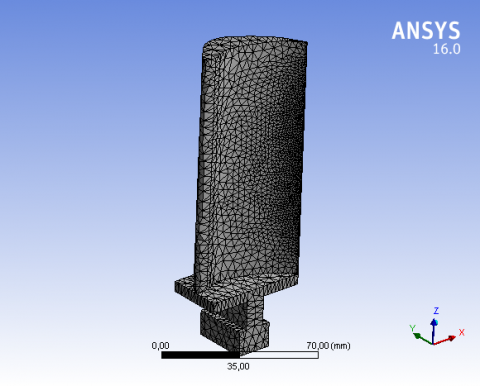Figure 2. Finite element model of turbine blade

7. Validations with Literature

Due to the absence of the experimental study for that is why we compared the results obtained with the results obtained by Ahmed . Structural analysis was carried out by applying the same limit conditions and mechanical forces selected materials for blade for validation. The desired comparison was made on the materials such as Aluminum 2024 Alloy, Stainless Steel Alloy and Titanium Alloy.

Figure 3 Show the displacement of the blade in the Titanium Alloy. the maximum displacement with a magnitude of 0.3915 mm.

## 3.png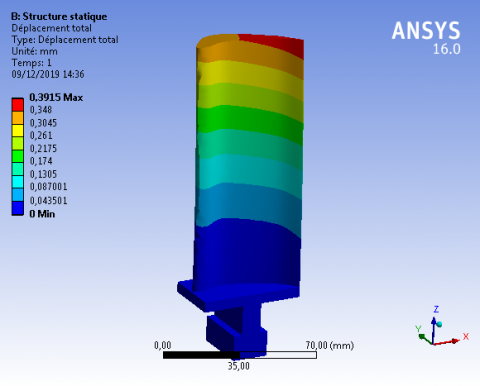Figure 3. Resultant displacements of titanium alloy

## 4.png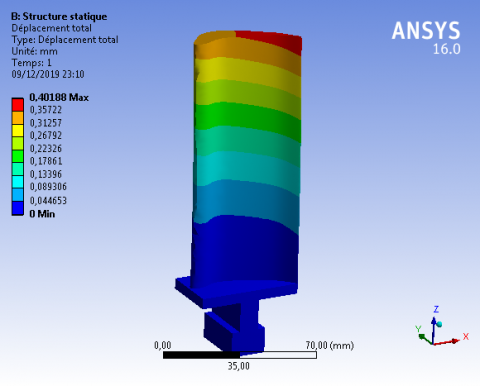Figure 4. Resultant displacements of stainless steel alloy

Figure 4 shows the displacement of the blade in the Stainless-Steel Alloy. The maximum displacement with a magnitude of 0.40188 mm.

## 5.png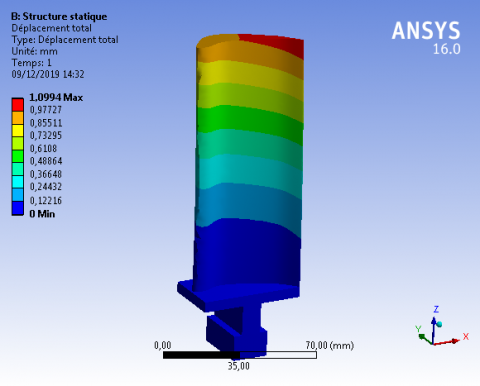Figure 5. Resultant displacements of aluminum 2024 alloy

Figure 5 Show the displacement of the blade in the Aluminum 2024 Alloy. the maximum displacement with a magnitude of 1.0994 mm.

## 6.pngFigure 6. Turbine blade materials as a function of displacements

To confirm the reliability of our numerical study, we compared the results of our attempt with the previous results.

The results obtained and published by Ahmed  are used to illustrate the validity of the attempt. Figure 6 Show the comparison between the displacements results for the (present numerical study and numerical studied by Ahmed . The results of displacement allowed us to note the conformity of the results given by the present numerical study and those obtained by Ahmed . It should be noted that the precision of the numerical study gives very acceptable results.

8. Results and Discussion

This work deals with modeling and analysis of the gas turbine blade were analyzed in ANSYS (Workbench 16.0) software. Different materials such as, Titanium Alloy, Stainless Steel Alloy, and Aluminum 2024 Alloy were compared to composite material.

In this case, we will study the static analysis of the blade in the composite material under the given mechanical loading and boundary conditions. The results of the analysis are discussed below.

## 7.png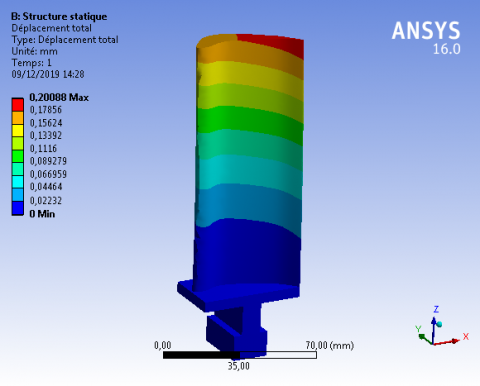Figure 7. Distribution of the displacements of a blade in composite material

## 8.pngFigure 8. Distribution of the principal elastic strains of a blade in composite material

Figure 7 Show the displacement of the blade in the composite material. the maximum displacement with a magnitude of 0.20088 mm at the ends of the blade and the minimum displacement at the foot of the blade.

Figure 8 shows the maximum principal strains of 0.0015036 occur at the root section and on the pressure side of the gas turbine blade.

## 9.pngFigure 9. Direction of path

## 10.pngFigure 10. Comparing the displacements (for 4 materials) according to the length of the blade

## 11.png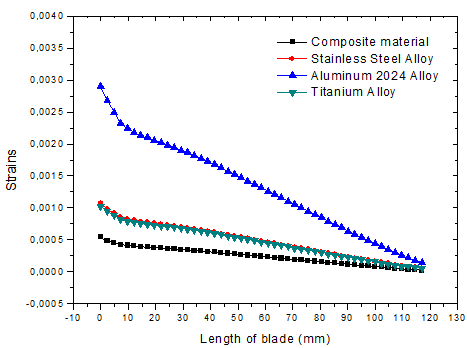Figure 11. Comparing the strains (for 4 materials) according to the length of the blade

## 12.png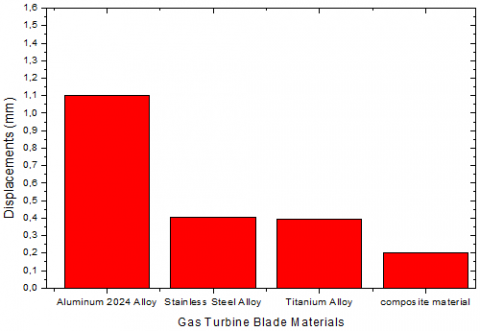Figure 12. Turbine blade materials according to displacements

The results giving displacements and strains of blade in different materials we will plot according to the continuous path between point 1 and point 2 shown on the Figure 9.

Figure 10 shows the evolution of the displacements as a function of the blade length. It can be noted that the displacement of the composite blade gives better results in comparison with other materials. It is also observed that the maximum value of the displacement is localized in the blade of Aluminum 2024 Alloy material at 1.0416 mm, while the minimum value is found in the composite blade which at 0.19042 mm.

Figure 11 shows the evolution of the strains as a function of the blade length. It can be noted that the strains of the composite blade give better results in comparison with other materials. It is also observed that the maximum value of the strain is localized in the blade of Aluminum 2024 Alloy material at 0.0028978 while the minimum value is found in the composite blade which at 0.0004139.

Figure 12 shows the variation of displacements distribution for different materials of turbine blade. It is noted that the displacements are varying as the radial distance increases, the maximum value for Aluminum 2024 Alloy and the minimum value for composite.

8.2 Thermomechanical analysis of turbine blade

## 13.png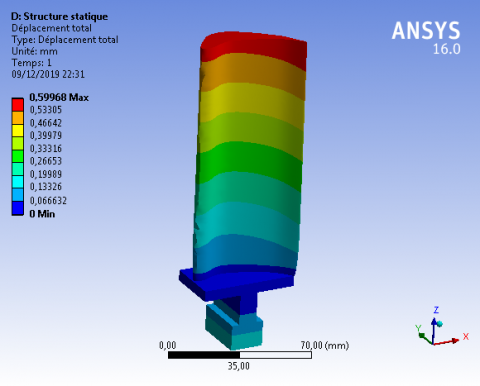Figure 13. Distribution of the displacements of a blade in composite material

## 14.png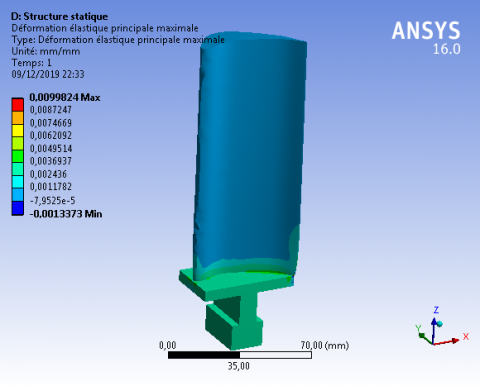Figure 14. Distribution of the principal elastic strains of a blade in composite material

In this case, we will study the thermomechanical behavior of the blade under the same loading conditions and boundaries previously defined. under the influence of temperature T= 800℃.

A summary of the results obtained from the thermal analysis of the same previous materials.

Figure 13 shows displacements produced in the turbine blade due to action of centrifugal forces and temperature. It is observed that the maximum deformation of 0.59968 mm occurs at the tip section of turbine blade material and the minimum occurs at the root section. There was no evidence of rubbing between tip of the turbine blade and casing indicating elongation is within the limit.

Figure 14 shows the Strain distribution in the turbine blade due to action of centrifugal forces and temperature. It is observed that the maximum strain of 0.0099824 occurs at the root section and on the pressure side of gas turbine blade. Minimum stress occurs at the tip section pressure side of gas turbine blade.

## 15.png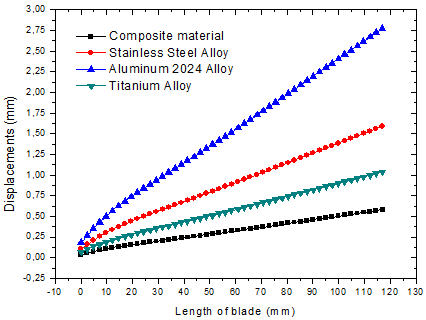Figure 15. Comparing the displacements (for 4 materials) according to the length of the blade

Figure 15 shows the evolution of the displacements as a function of the blade length. It can be noted that the displacement of the composite blade gives better results in comparison with other materials. It is also observed that the maximum value of the displacement is localized in the blade of Aluminum 2024 Alloy material at 2,7773 mm, while the minimum value is found in the composite blade which at 0,58044 mm.

## 16.png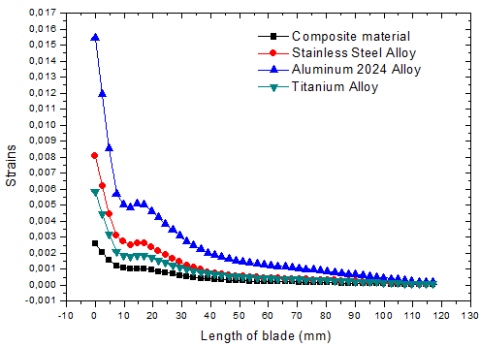Figure 16. Comparing the principal elastic strains (for 4 materials) according to the length of the blade

Figure 16 shows the evolution of the strains as a function of the blade length. It can be noted that the strains of the composite blade give better results in comparison with other materials. It is also observed that the maximum value of the strain is localized in the blade of Aluminum 2024 Alloy material at 0.015668, while the minimum value is found in the composite blade which at 0.0025769.

8.3 Analysis of turbine blade at various speeds

In this case, we will compare the same materials under temperature (T=800°C) with the change in the rotational speed value with three values: 4000 rpm, 6000 rpm, and 9000 rpm. Results on displacement and strain are shown in Tables 5, 6 respectively.

Table 5. Displacements of turbine blades made of different materials at various speeds

 Materials Displacements (mm) 4000(RPM) 6000(RPM) 9000(RPM) Composite material 0.52029 0.52041 0.52071 Titanium Alloy 0.91156 0.91195 0.91291 Stainless Steel Alloy 0.4927 1.4934 1.4951 Aluminum 2024 Alloy 2.4133 2.4139 2.4153

Table 6. Strains of turbine blades made of different materials at various speeds

 Materials Strains (mm) 4000(RPM) 6000(RPM) 9000(RPM) Composite material 0.01069 0.010684 0.01067 Titanium Alloy 0.020138 0.020115 0.020062 Stainless Steel Alloy 0.031627 0.03159 0.031508 Aluminum 2024 Alloy 0.053339 0.053301 0.053215

The results giving displacements and strains of blade in different materials shown in the Figures 17, 18.

## 17.png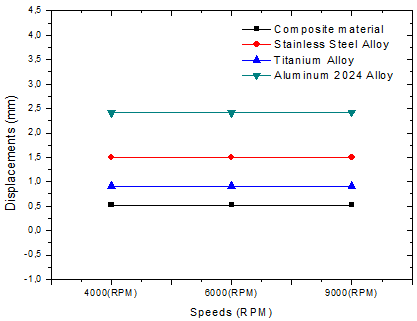Figure 17. Total displacements induced in turbine blades made of different materials at various speeds

## 18.png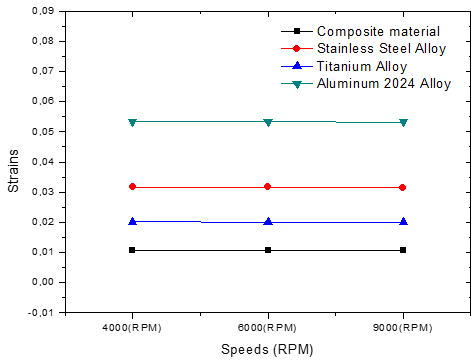Figure 18. Strains induced in turbine blades made of different materials at various speeds

The Thermomechanical analysis of turbine Blades with different rotational speeds 4000, 6000 and 9000 rpm’s by specifying structural and thermal loads with the objective of know the preferred material for the best performance. Based on the results obtained from the Ansys software the graphs, Figure 17 (Total displacement vs Rotation), Figure 18 (Strain vs Rotation) were plotted. The displacement and strains are proportionally increasing with an increase in rotation (RPM). this study it is concluded that the composite material should be used as turbine blade materials in the case of fixed or variable velocity because the displacement and strain is the minimum in the materials used in the comparison.

9. Conclusion

The choice of optimized composite material, significantly reduces the deformations along the fin and therefore locally reduces micro cracking causing wear flaking. In order to achieve the desired results, we completed the establishment of a research protocol in a predefined order:

1. A critical study on thermo-mechanical behavior in relation to the wear by flaking of gas turbine blades (problematic) for different materials.
2. A broad literature review on new materials (composites) and technical means to integrate them into the design and manufacture of turbine blades in general. The choice of a couple (reinforcement /matrix) is Carbon / ceramic after an optimization study to choose the optimum composition (fiber volume fraction vf=0.4).
3. Successive studies of simulations carried out on a real geometrical model (blade) of composite materials compared with the same model in super alloys to define essentially the displacements of the same point for the different materials and the local deformations.
4. The obtained results have showed a clear improvement of the fin stiffness and a reduction of the displacement of the extreme point of the blade. The local deformations are reduced. These results allow us to conclude that the cracking by scaling will be reduced significantly.
5. The optimization of a composite material (carbon/ceramic) in the design of turbojet blades has confirmed that the carbon/ceramic torque is very well adapted to an optimum thermo-mechanical operation. Increased stiffness, local reduction of deformations, reduction of micro cracking, and reduction of flaking and therefore increased life time can be achieved.
Acknowledgments

The authors like to thank the Algerian general direction of research (DGRSDT) for their financial support and the head of mechanical engineering laboratory at the University of Biskra for technical assistance.

Nomenclature
 E Young’s modulus of matrix, GPa Ef Young’s modulus of fiber, GPa EL Elastic modulus in the longitudinal direction, GPa Em Young’s modulus of matrix, GPa Emàt Young’s modulus of the mast reinforcement, GPa ET Elastic modulus in the transverse direction, GPa Fa Axial force N Fc Centrifugal force N Ft Tangential force N GL Shear modulus in longitudinal direction, GPa $G_{\text {mât }}$ Shear modulus of the mast reinforcement GT Shear modulus transverse direction, GPa L Length mm Vf Fiber volume fraction Vm Matrix volume fraction α Coefficient of thermal expansion, $10^{-6}\left({ }^{\circ} \mathrm{C}^{-1}\right)$ αL Thermal expansion in the longitudinal direction, $10^{-6}\left({ }^{\circ} \mathrm{C}^{-1}\right)$ αT thermal expansion in the Transverse direction, $10^{-6}\left({ }^{\circ} \mathrm{C}^{-1}\right)$ μ Poisson’s ratio μL Poisson’s ratio in the longitudinal direction $\mu_{\mathrm{mât}}$ Poisson’sratio of the mast reinforcement μT Poisson’s ratio in the Transverse direction $\rho$ Density [Kg/m3] $\delta$ Yield strength
References

 Jabbar, A.A., Rai, A.K., Reedy, P.R., Dakhil, M.H. (2014). Design and analysis of gas turbine rotor blade using finite element method. International Journal of Mechanical and Production, 4(1): 91-112.

 Rani, S., Agrawal, A.K., Rastogi, V. (2017). Failure analysis of a first stage IN738 gas turbine blade tip cracking in a thermal power plant. Case Studies in Engineering Failure Analysis, 8: 1-10. https://doi.org/10.1016/j.csefa.2016.11.002

 Ikpe, A., Oghenefejiro, E.O., Ariavie, G. (2018). Thermo-structural analysis of first stage gas turbine rotor blade materials for optimum service performance. International Journal of Engineering and Applied Sciences, 10(2): 118-130. https://doi.org/10.24107/ijeas.447650

 Rakotomalala, N. (2014). Simulation numérique de l’écaillage des barrières thermiques avec couplage thermo-mécanique (Doctoral dissertation, Paris, ENMP).

 Gurajarapu, N., Rao, V.N.B., Kumar, I.N. (2014). Selection of a suitable material and failure investigation on a turbine blade of marine gas turbine engine using reverse engineering and FEA techniques. International Journal of u-and e-Service, Science and Technology, 7(6): 297-308. https://doi.org/10.14257/ijunesst.2014.7.6.26

 Köster, A. (1997). Fatigue thermique d'un alliage pour aubes de turbopompe astronautique: le Superwaspaloy (Doctoral dissertation, Ecole Nationale Supérieure des Mines de Paris). https://www.researchgate.net/publication/46572302

 Théry, P.Y. (2007). Adhérence de barrières thermiques pour aube de turbine avec couche de liaison β-(Ni, Pt) Al ou β-NiAl (Zr) (Doctoral dissertation, Université Joseph-Fourier-Grenoble I). https://tel.archives-ouvertes.fr/tel-00258692

 Millecamps, A. (2010). Interaction aube-carter: contribution de l’usure de l’abradable et de la thermomécanique sur la dynamique d’aube (Doctoral dissertation, Lille 1). https://tel.archives-ouvertes.fr/tel-01200536

 Prasad, R.D.V., Raju, G.N., Rao, M.S., Rao, N.V. (2013). Steady state thermal & structural analysis of gas turbine blade cooling system. International Journal of Engineering Research & Technology (IJERT), 2(1).

 Krishnakanth, P.V., Raju, G.N., Prasad, R.D.V., Saisrinu, R. (2013). Structural and thermal analysis of gas turbine blade by using FEM. International Journal of Scientific Research Engineering and Technology, 2(2): 60-65.

 Prevost, M.A. (2007). Etude de nouvelles céramiques pour barrière thermique (Doctoral dissertation, Université Pierre et Marie Curie-Paris VI). https://tel.archives-ouvertes.fr/tel-00809130

 Gay, D. (2005). Matériaux composites. 5e édition.

 Mallick, P.K. (2007). Fiber-Reinforced Composites: Materials, Manufacturing, and Design. CRC Press. http://dx.doi.org/10.1201/9781420005981

 Berthelot, J.M. (2010). Mécanique des matériaux et structures composites. Institut Supérieur des Matériaux et Mécaniques Avancés.